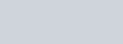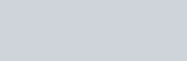# What is Logic Gates? and What are Different types of Logic Gates?Are you looking for the Logic Gates? So today we will study the Complete details on Logic Gates-(NOT, OR, AND, NOR, NAND, X-OR, X-NOR GATE), PDF. Here you will get the articles of Mechanical Engineering in brief with some key points and you will get to know an enormous amount of knowledge from It. So If you find this articles helpful, please let us know in the comment box, either if any correction required too, also let us know in the comment box.

## Logic Gates:

### Definition:

A logic gate is a circuit with one or more input voltages but only one output voltages.

logic gates are fundamental building blocks of the digital system. NOT, OR, and AND Gates are the basic types of gates.

The interconnection of gates to perform a variety of logical operation is called logic design. A truth table lists all possible combination of inputs and the corresponding outputs. All logic gates obey their truth table.

## NOT GATE:

A NOT gate produces an output that is the complement of the input. It has only one input signal.

It produces a 1 output when the input is 0. and

It produces a 0 output when the input is 1.

The four commonly used methods of expressing the NOT operation as shown in the figure.

In the English languageOutput Y is the complement of Input A
As a Boolean expressionY=Ā (“-” indicates NOT symbol)
As a symbolas a truth table
 A Y 0 1 1 0

The schematic diagram shows the idea of the NOT gate using a switch. The lamp (Y) will glow when the switch (A) is open. The lamp (Y) will not glow when the switch (A) is closed.Logic Gates

The two possible switch position is shown below. The truth table shows that NOT function of the switch and lamp circuit. The output of the NOT  circuit will be enabled (lamp lit) when the input switch is open.

 Input switch A Output light Y Open On Closed Off

Preparing for Competitive Exam? If Yes, then must go for this book:

The most valuable and trusted Mechanical Objective book by R S Khurmi 5000+ Objective.

## OR GATE:

An OR gate is sometimes called the “any or all gate”. It has 2 or more input signals.

It produces a 1 output of any or all inputs are 1.

It produces a 0 output when all inputs are 0.

An OR gate corresponds to the action of parallel switches for the input.

The below table shows the four commonly used methods for expressing the OR operation.

In the English languageInput A is ORed with input B to yield output Y
As a Boolean expressionA + B = Y  (‘+’ Indicates OR symbol)
As a symbolas a truth table
 A B Y 0 0 0 0 1 1 1 0 1 1 1 1

The schematics diagram shows the idea of the OR gates using switches.

The lamp (Y) will glow when either switch A or switch B is closed.

The lamp will also glow when both switches A and B are closed.

The lamp will not glow when both switches A and B are open.

###All the possible switch combination are shown.

Switch Combination:

 Input switches Output Light A B Y Open Open Off Open Closed On Closed Open On Closed Closed On

## AND GATE:

An AND gate is sometimes called the “all or nothing gate”. It has 2 or more input signals.

It produces a 1 output only when all inputs are 1. Otherwise, it produces a 0 output (nothing).

In other words, it produces a 0 output when any input is 0.

An AND gate corresponds to the action of series switches for the input.

The below table shows the four commonly used methods for expressing the AND operation.

In the English languageInput A is ANed with input B to yield output Y
As a Boolean expressionA.B = Y  (‘.’ Indicates AND symbol)
As a symbolas a truth table
 A B Y 0 0 0 0 1 0 1 0 0 1 1 1It shows the idea of the AND gate using switches.

The lamp (Y) will light only when both input switches (A and B) are closed.

All other possible combinations for switches A and B are shown in the below table.

Switch Combination:

 Input switches Output Light A B Y Open Open Off Open Closed Off Closed Open Off Closed Closed On

## NOR GATE:

A NOR gate represents an OR gate followed by an inverter. It has 2 or more input signals.

It produces a 1 when all the inputs are 0.

It produces a 0 output when any or all of the inputs are 1.

It therefore also acts as a negative (bubbled) AND gate.

The below table shows the four commonly used methods for expressing the NAND operation.

In the English languageInput A is NORed with input B to yield output Y
As a Boolean expressionA + B = Y  (‘+’ Indicates OR symbol)
As a symbolas a truth table
 A B Y 0 0 1 0 1 0 1 0 0 1 1 0

## NAND GATE:

A NAND gate presents an AND gate followed by an inverter. It has 2 or more input signals.

It produces a 0 output only when all the inputs are 1.

It produces a 1 output when any of the inputs is 0.

De Mergon’s second theorem says that the NAND gate is equivalent to a negative (bubbled) OR gate.

The below table shows the four commonly used methods for expressing the NAND operation.

In the English languageInput A is Nanded with input B to yield output Y
As a Boolean expressionAs a symbolas a truth table
 A B Y 0 0 1 0 1 1 1 0 1 1 1 0

Preparing for Competitive Exam? If Yes, then must go for this book:

The most valuable and trusted Mechanical Objective book by R S Khurmi 5000+ Objective.

## X-OR GATE:

An exclusive-OR (X-OR) gate is an anti-coincidence gate. It produces a 1 output only when the odd number of 1 is present at a time.

A 2-input X-OR gate is an inequality detector.

It produces a 1 output only when its two inputs are not equal i.e when one input is 1 or 0. An X-NOR gate can be used as a controlled inverter by connecting one input terminal to logic 1 and feeding the signal to be inverted to the other terminal.

The below table shows the four commonly used methods for expressing the X-OR operation.

In the English languageInput A and input B are X-ORed Yielding output Y
As a Boolean expressionAs a symbolas a truth table
 A B Y 0 0 1 0 1 0 1 0 0 1 1 1

## X-NOR GATE:

An exclusive-NOR (X-NOR) gate is a coincidence gate. It produces a 1 output only when even number of 1 is present at the input.

A 2-input X-NOR gate is an equality detector.

It produces a 1 output only when its two inputs are equal i.e when both inputs are 1 or 0. An X-NOR gate can be used as a controlled inverter by connecting one input terminal to logic 0 and feeding the signal to be inverted to the other terminal.

The below table shows the four commonly used methods for expressing the X-NOR operation.

In the English languageInput A and input B are XNORed Yielding output Y
As a Boolean expressionAs a symbolas a truth table
 A B Y 0 0 1 0 1 0 1 0 0 1 1 1